NEET  >  Test: Valence Bond Theory, Effective Atomic Number & Magnetic Properties

# Test: Valence Bond Theory, Effective Atomic Number & Magnetic Properties

Test Description

## 25 Questions MCQ Test Topic-wise MCQ Tests for NEET | Test: Valence Bond Theory, Effective Atomic Number & Magnetic Properties

Test: Valence Bond Theory, Effective Atomic Number & Magnetic Properties for NEET 2022 is part of Topic-wise MCQ Tests for NEET preparation. The Test: Valence Bond Theory, Effective Atomic Number & Magnetic Properties questions and answers have been prepared according to the NEET exam syllabus.The Test: Valence Bond Theory, Effective Atomic Number & Magnetic Properties MCQs are made for NEET 2022 Exam. Find important definitions, questions, notes, meanings, examples, exercises, MCQs and online tests for Test: Valence Bond Theory, Effective Atomic Number & Magnetic Properties below.
Solutions of Test: Valence Bond Theory, Effective Atomic Number & Magnetic Properties questions in English are available as part of our Topic-wise MCQ Tests for NEET for NEET & Test: Valence Bond Theory, Effective Atomic Number & Magnetic Properties solutions in Hindi for Topic-wise MCQ Tests for NEET course. Download more important topics, notes, lectures and mock test series for NEET Exam by signing up for free. Attempt Test: Valence Bond Theory, Effective Atomic Number & Magnetic Properties | 25 questions in 35 minutes | Mock test for NEET preparation | Free important questions MCQ to study Topic-wise MCQ Tests for NEET for NEET Exam | Download free PDF with solutions
 1 Crore+ students have signed up on EduRev. Have you?
Test: Valence Bond Theory, Effective Atomic Number & Magnetic Properties - Question 1

### Only One Option Correct Type Direction (Q. Nos. 1- 10) This section contains 10 multiple choice questions. Each question has four choices (a), (b), (c) and (d), out of which ONLY ONE is correct. Q.  Which one of the following is wrongly matched?

Detailed Solution for Test: Valence Bond Theory, Effective Atomic Number & Magnetic Properties - Question 1

Due to strong ligand, this is inner orbital complex and the hybridisation of Fe is d2sp3.

Test: Valence Bond Theory, Effective Atomic Number & Magnetic Properties - Question 2

### In which of the following the central atom has sp3d2-hybridisation?

Detailed Solution for Test: Valence Bond Theory, Effective Atomic Number & Magnetic Properties - Question 2

Since, F- ion is a weak ligand hence, pairing of electrons does not occur and outer orbital complex is formed in [CoF6]3-.

Test: Valence Bond Theory, Effective Atomic Number & Magnetic Properties - Question 3

### Which is the diamagnetic?

Detailed Solution for Test: Valence Bond Theory, Effective Atomic Number & Magnetic Properties - Question 3

[Ni(CN)4]2- in this, Ni has dsp2 hybridisation with square planar geometry and it is diamagnetic.

Test: Valence Bond Theory, Effective Atomic Number & Magnetic Properties - Question 4

A magnetic moment of 1.73 BM will be shown by one among following.

Detailed Solution for Test: Valence Bond Theory, Effective Atomic Number & Magnetic Properties - Question 4

A magnetic moment of 1.73 BM corresponds to one unpaired electron. Among the given, (a) and (b) are diamagnetic and (c) is paramagnetic with 3 unpaired electrons (3d7 configuration).

Test: Valence Bond Theory, Effective Atomic Number & Magnetic Properties - Question 5

Which one of the following complex species does not involve inner orbital hybridisation?

Detailed Solution for Test: Valence Bond Theory, Effective Atomic Number & Magnetic Properties - Question 5

F- ion is weak ligand hence, pairing of electrons does not occur and outer orbital complex is formed in [CoF6]3-.

Test: Valence Bond Theory, Effective Atomic Number & Magnetic Properties - Question 6

Which of the following complex has zero magnetic moment (spin only)?

Detailed Solution for Test: Valence Bond Theory, Effective Atomic Number & Magnetic Properties - Question 6

The potassium ferrocyanide, K4 [Fe(CN)6] due to strong ligand pairing of electrons occur and it has no unpaired electrons.

Test: Valence Bond Theory, Effective Atomic Number & Magnetic Properties - Question 7

The number of unpaired electrons calculated in [Co(NH3)6]3+ and [CoF6]3- are

Detailed Solution for Test: Valence Bond Theory, Effective Atomic Number & Magnetic Properties - Question 7

In [Co(NH3)6]3+ due to pairing cobalt configuration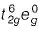and it is diamagnetic.
In [CoF6]3- , F- being weak ligand pairing does not occur and Co3+ ion has configuration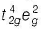. It has 4 unpaired electrons.

Test: Valence Bond Theory, Effective Atomic Number & Magnetic Properties - Question 8

The tetrachloro complexes of Ni(ll) and Pd(ll) respectively are (atomic number of Ni and Pd are 28 and 46 respectively).

Detailed Solution for Test: Valence Bond Theory, Effective Atomic Number & Magnetic Properties - Question 8

ln [NiCI4]2- , Ni has sp3 hybridisation with 2 unpaired electrons, i.e. paramagnetic.
In [PdCI4]2- Pd has dsp2 hybridisation and it has no unpaired electrons, i.e. diamagnetic.

Test: Valence Bond Theory, Effective Atomic Number & Magnetic Properties - Question 9

Which one of the following is an inner orbital complex as well as diamagnetic in behaviour? (Atomic number, Zn = 30, Cr= 24, Co = 27, Ni = 28)

Detailed Solution for Test: Valence Bond Theory, Effective Atomic Number & Magnetic Properties - Question 9

Zn and Ni always give outer orbital complexes with coordination number 6.
[Co(NH3)6]3+ is diamagnetic complex.
[Cr(NH3)6]3+ is param agnetic with 3d3 configuration.

Test: Valence Bond Theory, Effective Atomic Number & Magnetic Properties - Question 10

The pair of compounds having the same hybridisation for the central atom is

Detailed Solution for Test: Valence Bond Theory, Effective Atomic Number & Magnetic Properties - Question 10

[Co(NH3)6]3+ : For this complex action the 6 valance electrons of cobalt will occupy the 3d orbital, whereas the 6 ligands will occupy 3d,4s and 4p orbitals in d2sp3 hybridisation.

[Co(H2O)6]3+ : This intermixing is based on quantum mechanics. Transition metals may exhibit d2sp3 hybridization where the d orbitals are from the 3d and the s and p orbitals are the 4s and 3d. it acts as strong ligand when metal ion is in +3 oxidation for metals cobalt.

*Multiple options can be correct
Test: Valence Bond Theory, Effective Atomic Number & Magnetic Properties - Question 11

One or More than One Options Correct Type

Direction (Q. Nos. 11-15) This section contains 5 multiple choice questions. Each question has four choices (a), (b), (c) and (d), out of which ONE or MORE THAN ONE are correct.

Q.

The formation of complex which involves d-orbitals of outer shell are called

Detailed Solution for Test: Valence Bond Theory, Effective Atomic Number & Magnetic Properties - Question 11

The complex which involves d-orbitals of outer shell are called high spin complex as well as outer orbital complex.

*Multiple options can be correct
Test: Valence Bond Theory, Effective Atomic Number & Magnetic Properties - Question 12

sp3-hybridisation is found in

Detailed Solution for Test: Valence Bond Theory, Effective Atomic Number & Magnetic Properties - Question 12

[ZnCI4]2-, [CuCI4]2- and [Ni(CO)4] have sp3 hybridisation and all three contains weak field ligands.

*Multiple options can be correct
Test: Valence Bond Theory, Effective Atomic Number & Magnetic Properties - Question 13

Regarding the complex [Co(H2O)6]2- , correct statement(s) is/are

Detailed Solution for Test: Valence Bond Theory, Effective Atomic Number & Magnetic Properties - Question 13

Since, F- ion is weak ligand it forms outer orbital complex arid it has 3d7 configuration with 3 unpaired electrons.

*Multiple options can be correct
Test: Valence Bond Theory, Effective Atomic Number & Magnetic Properties - Question 14

For which the EAN value is equal to the atomic number of a noble gas?

Detailed Solution for Test: Valence Bond Theory, Effective Atomic Number & Magnetic Properties - Question 14

K2[Hgl4] In this EAN = 80 - 2 + 8 = 86 (Equal to noble gas, radon).
[Pd(NH3)CI2] In this EAN = 46 - 2 + 8 = 52 (Not equal to noble gas, xenon)
[Cdl4]2- In this EAN = 48 - 2 + 8 = 54 (Equal to noble gas, xenon)
Co2(CO)8 In this EAN = Electrons from Co-atom (27) + electrons from 4 CO molecules (8) + one electron from Co— Co bond (1) = 36 (Equal to noble gas krypton)

*Multiple options can be correct
Test: Valence Bond Theory, Effective Atomic Number & Magnetic Properties - Question 15

[Fe(H2O)6]2+ and [Fe(CN)6]4- differ in

Detailed Solution for Test: Valence Bond Theory, Effective Atomic Number & Magnetic Properties - Question 15

[Fe(H2O)6]2+ and [Fe(CN)6]4- have different hybridisation, magnetic moment and colour also.

Test: Valence Bond Theory, Effective Atomic Number & Magnetic Properties - Question 16

Comprehension Type

Direction (Q. Nos. 16 and 17) This section contains a paragraph, describing theory, experiments, data, etc. Two questions related to the paragraph have been given. Each question has only one correct answer among the four given options (a), (b), (c) and (d).

Passage

When crystals of CuSO4 . 4NH3 are dissolved in water, there is hardly any evidence for the presence of Cu2+ ions or ammonia molecules. A new ion [Cu(NH3)4]2+ is furnished in which ammonia molecules are directly linked with the metal ion. Similarly, aqueous solution of Fe(CN)2 .4KCNdoes not give tests of Fe2+ and CN- ions but give test for new ion [Fe(CN)6]4- called ferrocyanide ion.

Q.

The hybridisation and geometry and magnetic property of [Cu(NH3)4]2+ ion are

Detailed Solution for Test: Valence Bond Theory, Effective Atomic Number & Magnetic Properties - Question 16

Cu(Z = 29) copper configuration (3d104s1)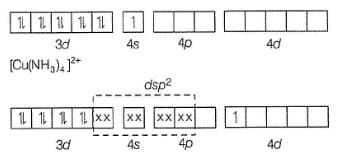Hence, [Cu(NH3)4]2+ has square planar geometry and 1 unpaired electron in Ad orbital which is transferred from 3d orbital with magnetic moment 1.73 BM.

Test: Valence Bond Theory, Effective Atomic Number & Magnetic Properties - Question 17

When crystals of CuSO4 . 4NH3 are dissolved in water, there is hardly any evidence for the presence of Cu2+ ions or ammonia molecules. A new ion [Cu(NH3)4]2+ is furnished in which ammonia molecules are directly linked with the metal ion. Similarly, aqueous solution of Fe(CN)2 .4KCNdoes not give tests of Fe2+ and CN- ions but give test for new ion [Fe(CN)6]4- called ferrocyanide ion.

Q.

The hybridisation, geometry and magnetic property of [Fe(CN)6]4- are

Detailed Solution for Test: Valence Bond Theory, Effective Atomic Number & Magnetic Properties - Question 17

It is inner orbital complex, diamagnetic.

Test: Valence Bond Theory, Effective Atomic Number & Magnetic Properties - Question 18

Matching List Type

Direction (Q. Nos. 18 and 19) Choices for the correct combination of elements from Column I and Column II are given as options (a), (b), (c) and (d) out of which one is correct.

Q.

Match the Column I with Column II and mark the correct option from the codes given bleow.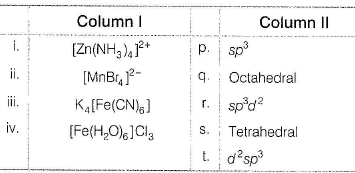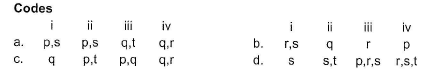Detailed Solution for Test: Valence Bond Theory, Effective Atomic Number & Magnetic Properties - Question 18

(i) → (p.s), (ii) → (p,s), (iii) → (q,t), (iv) → (q.r)

Test: Valence Bond Theory, Effective Atomic Number & Magnetic Properties - Question 19

Match the Column I with Column il and mark the correct option from the codes given bleow.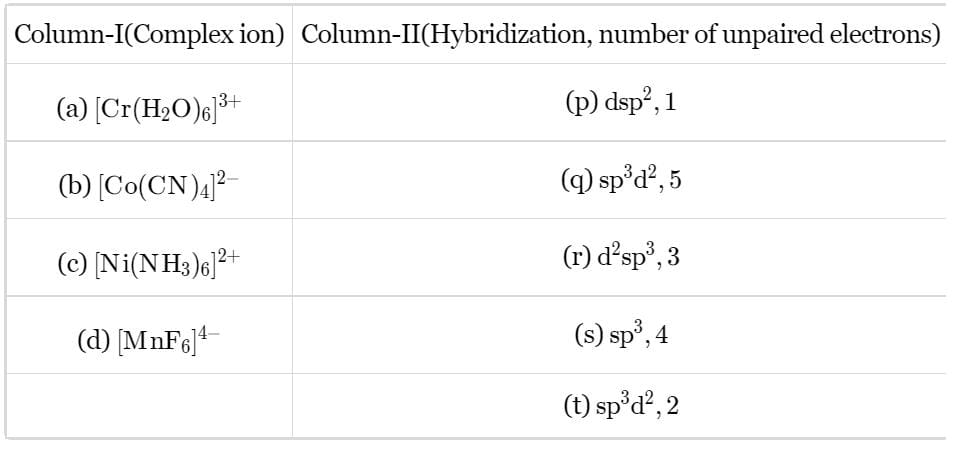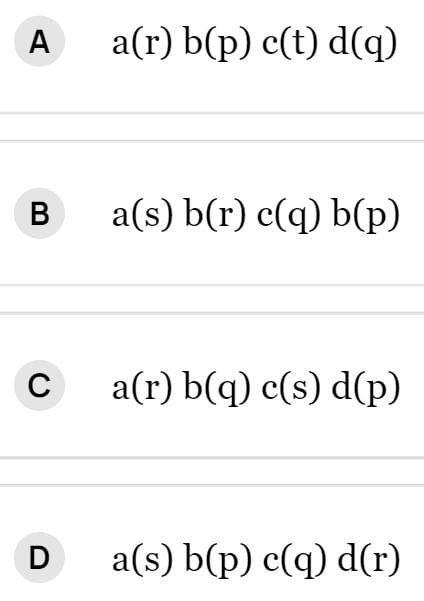Detailed Solution for Test: Valence Bond Theory, Effective Atomic Number & Magnetic Properties - Question 19

A → (iii) (b) B → (i) (c) C → (iv) (d) D → (ii)

a) Six empty orbitals (two d, one s and three p) are available, so whether the ligand is weak (H2​O)  the six orbitals hybridize to give six equivalent d2sp3 hybrid orbitals. Hence, in  [Cr(H2​O)6​]3+  ions chromium is in a state of d2sp3 hybridization.b)  CN is a strong ligand it makes the unpaired electrons of cobalt to pair up and occupies the space.

• The ligands occupy one d orbital, one s orbital and 2 p orbitals. Thus the hybridisation is “dsp2” with “square planar” geometry.
• It has 1 unpaired electron.

c) In [Ni(NH3)6]2+, Ni is in +2 state and has configuration 3d8. In presence of NH3, the 3d electrons do not pair up. The hybridization is sp3d2 forming an outer orbital complex.

It has been found that the complex has two unpaired electrons.

*Answer can only contain numeric values
Test: Valence Bond Theory, Effective Atomic Number & Magnetic Properties - Question 20

One Integer Value Correct Type

Direction (Q. Nos. 20-24) This section contains 5 questions. When worked out will result in an integer from 0 to 9 (both inclusive).

Q.

How many of the following species obey Sidgwick EAN rule?

K3[Fe(CN)6], [Ru(CO)5], [Cr(NH3)6] 3+, [Co(NH3)6]3+, [Ni(NH3)6]2+, [Fe(CO)5],[W(CO)6]

Detailed Solution for Test: Valence Bond Theory, Effective Atomic Number & Magnetic Properties - Question 20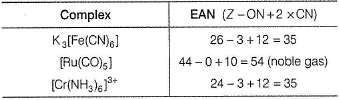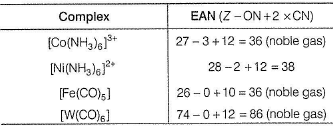*Answer can only contain numeric values
Test: Valence Bond Theory, Effective Atomic Number & Magnetic Properties - Question 21

How many of the following are diamagnetic?

[Zn(OH)4]2-,[Ni(NH3)6]2+, K4[Fe(CN)6], K3[Fe(CN)6], [Cu(NH3)4]2+,[PdBr4]2-, [Ni(CO)4], [CoF6] 3-

Detailed Solution for Test: Valence Bond Theory, Effective Atomic Number & Magnetic Properties - Question 21

Diamagnetic are
[Zn(OH)4]2-, K4[Fe(CN)6], [PdBr4]2-,[Ni(CO)4]

*Answer can only contain numeric values
Test: Valence Bond Theory, Effective Atomic Number & Magnetic Properties - Question 22

The number of unpaired electrons in the complex [Mn(acac)3] is

(Atomic number of Mn= 25)

Detailed Solution for Test: Valence Bond Theory, Effective Atomic Number & Magnetic Properties - Question 22

In this, Mn has +3 oxidation state. Mn3+ configuration = [Ar]3d4. This forms outer complex. Hence, unpaired electrons are 4.

*Answer can only contain numeric values
Test: Valence Bond Theory, Effective Atomic Number & Magnetic Properties - Question 23

The magnetic moment of [Ru(H2O)6]2+ corresponds to the presence of ...... unpaired electrons.

Detailed Solution for Test: Valence Bond Theory, Effective Atomic Number & Magnetic Properties - Question 23

R = [Kr]4d7 5s1 (in ground state)
In Ru[2+] => 4d6
=> (t2g)6 (eg)0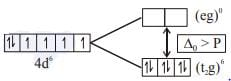Hence, no unpaired electron. Therefore, correct answer is zero.

*Answer can only contain numeric values
Test: Valence Bond Theory, Effective Atomic Number & Magnetic Properties - Question 24

The number of unpaired electrons in [Fe(dipy)3]2+ are

Detailed Solution for Test: Valence Bond Theory, Effective Atomic Number & Magnetic Properties - Question 24

In [Fe(dipy)3]2+ complex ion, Fe has 3d6 configuration. Due to pairing, this has no unpaired electrons.

Test: Valence Bond Theory, Effective Atomic Number & Magnetic Properties - Question 25

Statement Type

Direction (Q. No. 25) This section is based on Statement I and Statement II. Select the correct answer from the codes given below.

Q.

Statement I : Both [Ni(CN)4]2- and [NiCI4]2- have same shape and same magnetic behaviour.

Statement II : Both are square planar and diamagnetic

Detailed Solution for Test: Valence Bond Theory, Effective Atomic Number & Magnetic Properties - Question 25

Statement I [Ni(CN)4]2- is square planar and diamagnetic whereas [NiCI4]2- is tetrahedral and paramagnetic .
Statement II [Ni(CN)4]2- is square planar while [NiCI4]2- is tetrahedral.

## Topic-wise MCQ Tests for NEET

1493 tests
 Use Code STAYHOME200 and get INR 200 additional OFF Use Coupon Code
Information about Test: Valence Bond Theory, Effective Atomic Number & Magnetic Properties Page
In this test you can find the Exam questions for Test: Valence Bond Theory, Effective Atomic Number & Magnetic Properties solved & explained in the simplest way possible. Besides giving Questions and answers for Test: Valence Bond Theory, Effective Atomic Number & Magnetic Properties, EduRev gives you an ample number of Online tests for practice

## Topic-wise MCQ Tests for NEET

1493 tests

### How to Prepare for NEET

Read our guide to prepare for NEET which is created by Toppers & the best Teachers